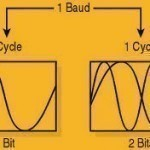•• # Symbol Rate

The symbol rate is the rate of state changes on a communications circuit.

If a circuit can carry two tones per second, the circuit has a symbol rate of two.

Circuits then use different modulation techniques to carry multiple bits per symbol.

If the circuit is limited to two different tones, the first tone can represent a 0 and the second tone can represent a 1. In this circuit, the symbol rate is the same as the bit rate.

If the circuit can carry four different tones, then the tones can be used to encode twice as many bits per symbol. In this circuit, the bit rate is now twice the symbol rate.Using more tones allows more bits per second (bps) to be squeezed out of every symbol, but this also requires higher quality circuits. If the circuit is not high enough quality, the number of retransmissions will cause the circuit to be slower than with a lower number of tones.

The choice of how many tones to use is determined by the modulation algorithm chosen. QPSK uses four tones, 8PSK uses eight tones.

The use of 4 tones is standard in the satellite world. In the cable television world, the higher quality transmission medium enables 64QAM modulation to be the standard.

Symbol Rate is abbreviated as SR.

The symbol rate is also known as the baud rate.